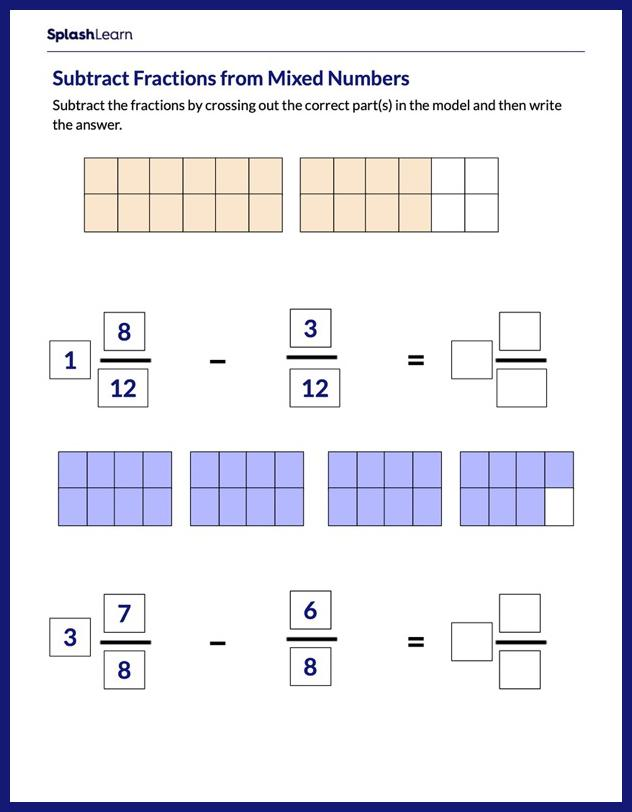# Subtract Fractions from Mixed Numbers Using Models Worksheet

Home > Subtract Fractions from Mixed Numbers Using ModelsBuild your child's subtraction of fractions skills with this worksheet. The worksheet challenges students to solve a set of problems on fractions. These engaging problems encourage them to apply their prior knowledge of the topic and subtract fractions from mixed numbers using area models. This worksheet will nudge your young mathematician toward mastery and develop their all round mathematical acumen.